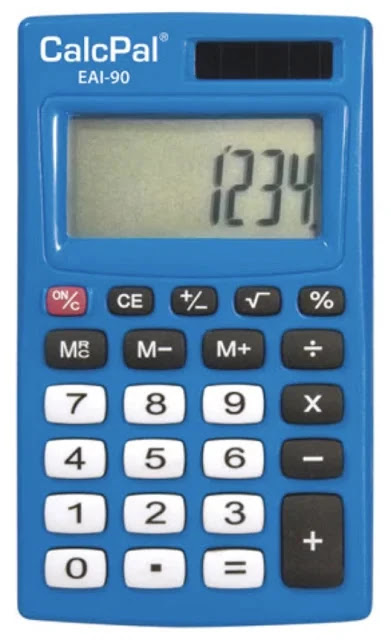### Creating a Simple Calculator in Scratch

Hello and Welcome to Scratch 101! In this tutorial we will be creating a simple four-function calculator! These four functions are addition (+), subtraction (-), multiplication (*) and division. Furthermore, these kinds of calculators will typically include a number pad with the values zero through nine.

Objective: To understand the mathematical operations within Scratch and how to combine these with user input/conditional statements.Example of a Four Function Calculator.
It would not be difficult to expand these principles to a project involving a scientific calculator since Scratch contains many of these advanced functions as well.

Graphic Template: The graphic template is located here although the only customization is the new background image.

Scripts:

Consider: What other functions would you wish to include in this list? What if you wanted to increase that you could work with? How would be do more than one operation at a time (i.e. without having to restart the program every time)? How could you represent a running list of operations continuously? How would you build in PEMDAS (Order of Operations) into expressions with multiple operations. Think about all of these question in the context of the list data type.

Wrap Up: If you are interested in more mathematics projects, feel free to consider the Fibonacci and Factorial projects in the 2.0. series. Furthermore, you could create quadratic, cubic or quartic equation solvers in Scratch or learn about your own mathematical concept and convert it to a programming exercise. Leveraging computer science with statistics specifically is an excellent endeavor because random number generators are great with sampling.